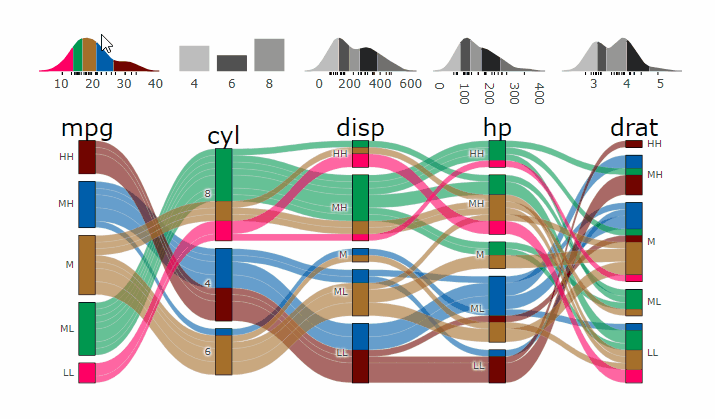`parcats 0.0.3` was released on CRAN. It is an htmlwidget providing bindings to the `plotly.js` parcats trace, which is not supported by the `plotly` R package. It also adds marginal histograms for numerical variables.demogif

# Better {shiny} Support

It now integrates better into `shiny` apps. There is a new function `parcats_demo()` which let’s you interactively explore all the different parameters of `easyalluvial::alluvial_wide()` and `parcats::parcats()`. You can see how the alluvial plot and the derived interactive parcats widget look like with different parameters.

demo

# Update {plotly.js}

In order to be 100% compatible with R `plotly`. `plotly.js` that is shipped with `parcats` has been upgraded to `v2.5.1`

# Parcats from Alluvial Plot

``````suppressPackageStartupMessages(require(tidyverse))
suppressPackageStartupMessages(require(easyalluvial))
suppressPackageStartupMessages(require(parcats))
suppressPackageStartupMessages(require(parsnip))``````
``````p = alluvial_wide(mtcars2, max_variables = 5)

parcats(p, marginal_histograms = TRUE, data_input = mtcars2)``````

# Partial Dependence Alluvial Plots

Machine Learning models operate in a multidimensional space and their response is hard to visualise. Model response and partial dependency plots attempt to visualise ML models in a two dimensional space. Using alluvial plots or parallel categories diagrams we can increase the number of dimensions.

``````df <- select(mtcars2, -ids)

m <- parsnip::rand_forest(mode = "regression") %>%
parsnip::set_engine("randomForest") %>%
parsnip::fit(disp ~ ., df)

p <- alluvial_model_response_parsnip(m, df, degree = 4, method = "pdp")``````
``## Getting partial dependence plot preditions. This can take a while. See easyalluvial::get_pdp_predictions() `Details` on how to use multiprocessing``
``parcats(p, marginal_histograms = TRUE, imp = TRUE, data_input = df)``